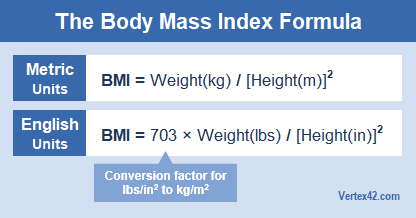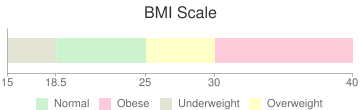With Vertex42's online BMI Calculator, you can either calculate your Body Mass Index based on height and weight, or you can enter your desired BMI and solve for your goal weight. It also shows the result within a BMI Scale. Check out our BMI Chart if you are looking for something you can print that will show BMI for multiple heights and weights.

How to Calculate BMI - The BMI FormulaBody Mass Index is defined as your weight divided by the square of a your height, using the metric units kg/m^2. The BMI formula does not apply to children (see the references below). You can calculate BMI using one of these BMI formulas:

• Metric Units: BMI = Weight[kg] / ( Height[m] x Height[m] )
• English Units: BMI = 703 x Weight[lb] / ( Height[in] x Height[in] )

Online BMI Calculator

BMI Calculator

 Height feet inches lbsReferences

Disclaimer: This calculator is for illustrative and educational purposes only. We do not guarantee the results or the applicability to your unique situation. You should seek advice from qualified professionals.

Follow Us On ...

Share: